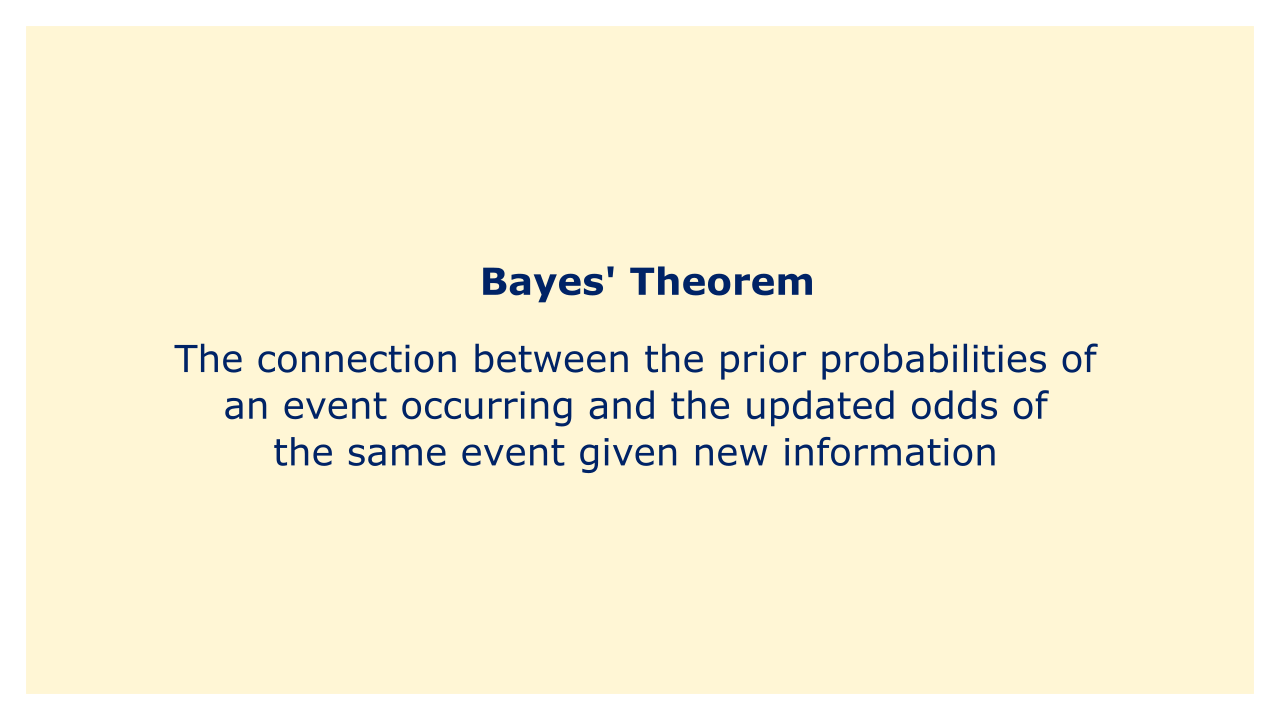# Bayes' TheoremImage: Moneybestpal.com

### A mathematical statement known as the Bayes Theorem explains the connection between the prior probabilities of an event occurring and the updated odds of the same event given new information. The theorem is named after Thomas Bayes, a statistician, and priest who lived in the 18th century, who first proposed it in his posthumous work, "An Essay towards Solving a Problem in the Doctrine of Chances."

The Bayes Theorem, in its most basic form, states that the likelihood of new information given an event is equal to the sum of the likelihood of the occurrence given new information and the prior probability of the event. The formula can be expressed mathematically as:

P(A | B) = P(B | A) * P(A) / P(B)

Where:
P(A | B) is the updated probability of event A occurring, given new information B
P(B | A) is the likelihood of observing new information B, given that event A has occurred
P(A) is the prior probability of event A occurring
P(B) is the prior probability of observing new information B

Natural language processing, machine learning, data analysis, and decision-making all make extensive use of Bayes' Theorem. When it comes to financing, Bayesian approaches are used to anticipate future values of financial variables, estimate the parameters of financial models, and measure the risk and uncertainty involved with investment choices.
Tags